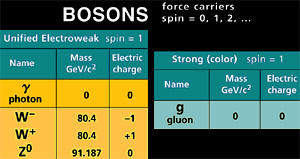A# bosonA boson is any particle with a spin that is an integer multiple (0, 1, 2, ...) of quantum units of angular momentum. Bosons, named by Paul Dirac in 1947 after the Indian physicist Satyendra Nath Bose, are the carrier particles of all fundamental interactions. A carrier particle is a boson associated with quantum excitations of the field corresponding to one of the basic forces of nature (see below).

Bosons do not obey the Pauli exclusion principle. This means there is no limit to the number of bosons that can occupy the same point in space at the same time; so, for example, there is no limit in theory to the brightness of a beam of light. Bosons are one of two main categories of particle in the Standard Model; the other main category is the fermions.

## Bosons as carrier particles

The bosons that serve as carrier particles for the four basic forces in nature – electromagnetism, the strong force, the weak force, and (in theory) gravity – are known collectively as gauge bosons. Their existence is demanded by a principle called gauge invariance.

The photon, the carrier of the electromagnetic force, is a massless, uncharged boson with spin 1. The W and Z bosons, also called intermediate vector bosons, mediate the weak force between leptons and in the decay of hadrons. The Z boson is electrically neutral, whereas the W boson comes in two forms: positively and negatively charged.

The gluon is the carrier particle of the strong interaction that holds quarks together. Gluons are electrically neutral and have spin 1. They are the equivalent in quantum chromodynamics of photons in quantum electrodynamics. Neither quarks nor gluons exist as isolated particles, but only as constituents of hadrons. The as-yet-undetected graviton, presumed to be associated with the gravitational force between masses, is theorised to have spin 2.

The existence of another type of boson, known as the Higgs boson, has been established from observations made at the Large Hadron Collider and the Tevatron beginning in 2012. The Higgs boson was hypothesized as the means by which all other particles in the Standard Model have the masses they do through what is called the Higgs field. The existence of the Higgs, which was first theorised in 1964, completes the Standard Model.